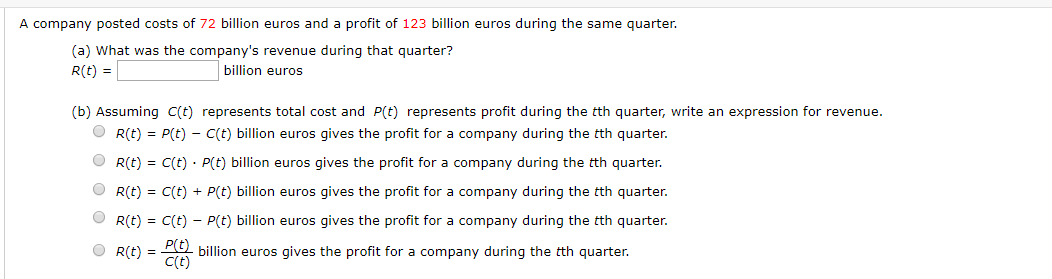# A company posted costs of 72 billion euros and a profit of 123 billion euros during the same quarter(a) What was the company's revenue during that quarter?billion eurosR(t)(b) Assuming C(t) represents total cost and P(t) represents profit during the tth quarter, write an expression for revenue.R(t)P(t)- C(t) billion euros gives the profit for a company during the tth quarter.C(t) P(t) billion euros gives the profit for a company during the tth quarter.R(t)R(t)C(t)+ P(t) billion euros gives the profit for a company during the tth quarter.R(t)C(t)P(t) billion euros gives the profit for a company during the tth quarter.billion euros gives the profit for a company during the tth quarter.C(t)R(t)

Question
1 viewshelp_outlineImage TranscriptioncloseA company posted costs of 72 billion euros and a profit of 123 billion euros during the same quarter (a) What was the company's revenue during that quarter? billion euros R(t) (b) Assuming C(t) represents total cost and P(t) represents profit during the tth quarter, write an expression for revenue. R(t) P(t)- C(t) billion euros gives the profit for a company during the tth quarter. C(t) P(t) billion euros gives the profit for a company during the tth quarter. R(t) R(t) C(t)+ P(t) billion euros gives the profit for a company during the tth quarter. R(t) C(t) P(t) billion euros gives the profit for a company during the tth quarter. billion euros gives the profit for a company during the tth quarter. C(t) R(t) fullscreen
check_circle

Step 1

(a)

Given information:

Total cost is 72 billion euros.

Profit is 123 billion euros.

To find the company’s revenue during the quarter, add the total cost and the net profit.

Step 2

(b)

Assume C(t) represents the total cost and P(t) represents profit during th...

### Want to see the full answer?

See Solution

#### Want to see this answer and more?

Solutions are written by subject experts who are available 24/7. Questions are typically answered within 1 hour.*

See Solution
*Response times may vary by subject and question.
Tagged in

### Other